Next:8.3.2 Prim's AlgorithmUp:8.3 Minimum-Cost Spanning TreesPrevious:8.3 Minimum-Cost Spanning Trees

## 8.3.1 MST Property

SupposeG = (V, E)is a connected graph with costs defined on all e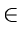E. Let U be some proper subset of V.

If (u, v) is an edge of lowest cost such that uUand vV - U, then there exists an MST that includes (u, v) as an edge. See Figure 8.5.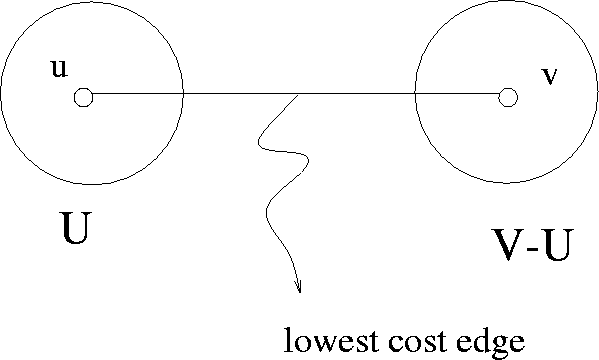Proof of MST Property

Suppose to the contrary that there is no MST for the graph Gwhich includes the edge (u, v).

Let T be any MST of G. By our assumption, T does not contain (u, v). Adding (u, v) to Twill introduce a cycle since T is a free tree. This cycle involves (u, v). Therefore there is a path fromv to u that does not pass through this edge. This means that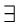another edge (u', v') in T such that u'Uandv'V - U. See Figure 8.6.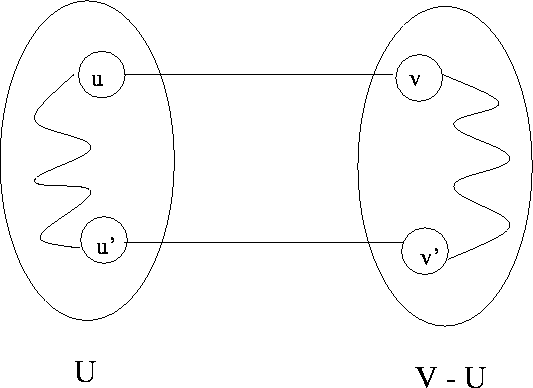Deleting edge (u', v') breaks the cycle and yields a spanning tree T' whose cost is certainly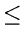that of T since C(u, v)C(u', v'). Thus we have constructed an MST that includes (u, v).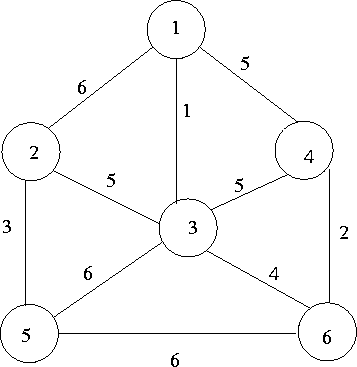To illustrate, consider the graph in Figure 8.7 and refer to Figures 8.8 and 8.9. Consider the sets:

 U = {1, 2, 5} V - U = {3, 4, 6}.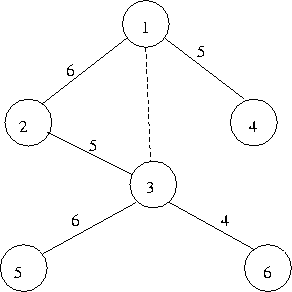Spanning tree with cost = 26. Now, least cost edge from U to V - U is (1,3).

By including (1,3) in the above spanning tree, a cycle will form (for example, 1-2-3-1). Let us replace the edge (2,3) by the edge (1,3).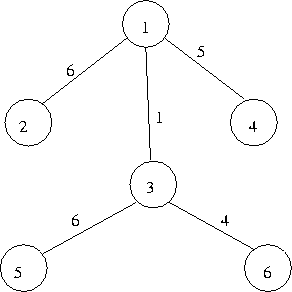This has yielded a ST with cost = 22

DEF.
A set of edges T in a connected graph promising if it can be extended to produce a minimal spanning tree for the graph.
• By definition, T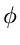is always promising since a weighted connected graph always has at least one MST.
• Also, if a promising set of edges T is a spanning tree, then it must be an MST.
Def: An edge is said to leave a given set of nodes if exactly one end of this edge is in the set.

MST Lemma: Let

• G = (V, E) be weighted connected graph
• U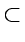V a strict subset of nodes in G
• T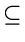E a promising set of edges in E such that no edge in T leaves U
• e a least cost edge that leaves U
Then the set of edgesT' = T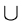{e} is promising.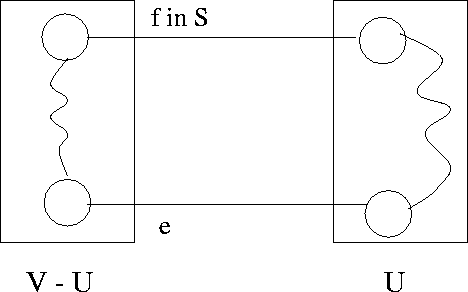Proof

Since T is promising, it can be extended to obtain an MST, says S. If eS, there is nothing to prove.

If eS, then we add edge e to S, we create exactly one cycle (since S is a spanning tree). In this cycle, since e leaves U there exists at least one other edge, f say, that also leaves U (otherwise the cycle could not close). See Figure 8.10.

If we now remove f, the cycle disappears and we obtain a new tree R that spans G.

Note thatR = (S{e}) - {f}

Also note that weight of eweight of f since e is a least cost edge leaving U.

Therefore R is also an MST and it includes edge e. Furthermore TR and so can be extended to the MST R. Thus T is a promising set of edges.Next:8.3.2 Prim's AlgorithmUp:8.3 Minimum-Cost Spanning TreesPrevious:8.3 Minimum-Cost Spanning Trees
eEL,CSA_Dept,IISc,Bangalore